First Law of Thermodynamics & Heat Capacities of a System

# First Law of Thermodynamics & Heat Capacities of a System - Notes | Study Physical Chemistry - Chemistry

 1 Crore+ students have signed up on EduRev. Have you?

First Law of Thermodynamics “The first law of thermodynamics states that energy can neither be created nor destroyed, although it can be transformed from one form to another.” This is first law of thermodynamics.

Internal Energy (U): Every substance is associated with definite amount of energy which depends upon its chemical nature as well as upon its temperature, pressure and volume. This energy is known as internal energy.

Relation between first law & internal energy: Let us a system is subjected to change of pressure and vo lume. Let the init ial state is A and final state is B represented and UA & UB be its init ial & final energy.
Then                 ΔU = UB - UA

Suppose on changing the system form A to B absorbs heat q from surroundings and also performs some work equal to ω. The absorption of heat raise the energy of system.
Then change in internal energy;
ΔU = UB - UA = q - ω

If in a given process, the quantity of heat transferred fro m the surroundings to the system is q and work done in process is w , then change in internal energy is
ΔU = q + ω ,

This is the statement of first law of thermodynamics.

i.e. when work done by system then expansion takes place and work is always negative.
When work done on the system takes place then compression takes place and work done is always positive.  When work done by the system takes place then expansion takes place and work done is always negative.

Enthalpy of the system: The quantity U + PV is known as the enthalpy of the system and is denoted by H. It represented the total energy of the system. Thus,

H = U + PV

Heat capacity of a system: The quantity of heat required to raise the temperature of the system from the lower to the higher temperature divided by the temperature difference is known as heat capacity. If mass of the system is one gram than it is specific heat capacity and if mass in mole than it is molar heat capacity. It is denoted by C.

C =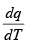The molar heat capacity of a gaseous system, determined at constant volume, is different from that determined at constant pressure.
In the former case, no external work is done by the system or on the system (i.e. ω = 0) since there is no change in volume. Hence, from the first law equation:
dq = dU – ω                  (ω = 0)
dq = dU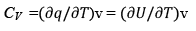for 1 mole
CV =(1/n) (∂U/∂T)v

for n mole

or                     dU = nCV dT

As constant pressure, there is change of volume and some work is done.  The work done is ω. Then from first law: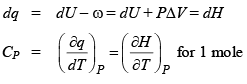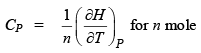dH = n CP dT

Relation between CP and CV in gaseous system Let entropy of the system is S and its value depends on temperature and pressure.
i.e. S is the funct ion of P & T.
S = S(P, T)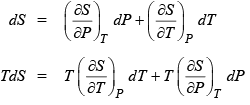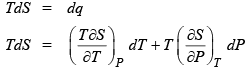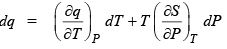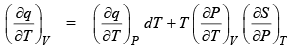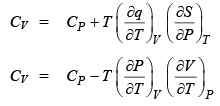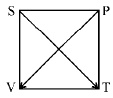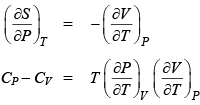Using this relationship we can find many relat ionships between CP & CV. These are given below:

(1)  CP – CV = R (for 1 mole of ideal gas).

(2)  CP – CV = R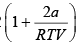(for 1 mole of real gas).

(3)  CP – CV =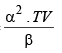(4)  CP - CV = TE α2V

E = elasticity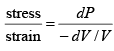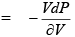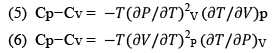The document First Law of Thermodynamics & Heat Capacities of a System - Notes | Study Physical Chemistry - Chemistry is a part of the Chemistry Course Physical Chemistry.
All you need of Chemistry at this link: Chemistry

## Physical Chemistry

85 videos|131 docs|67 tests
 Use Code STAYHOME200 and get INR 200 additional OFF

## Physical Chemistry

85 videos|131 docs|67 tests

Track your progress, build streaks, highlight & save important lessons and more!

,

,

,

,

,

,

,

,

,

,

,

,

,

,

,

,

,

,

,

,

,

;